# RAM Test

This project tests the memory access actions of a simple computer. The load instruction (Opcode 0x01) reads data from a specified memory address into the accumulator. The store instruction (Opcode 0x04) writes data from the accumulator into a specified memory address.

Memory can be examined in the simulator after running the program by clicking on the Logical Memories section in the left column of the simulation report tree list.### Example

```  C = A + B
```

If we assume that A is stored in address 0x10, B is in address 0x11, and the result C should be stored in 0x12, then we have the following program:

Assembly LanguageMachine Language
STORE C0412

## Architecture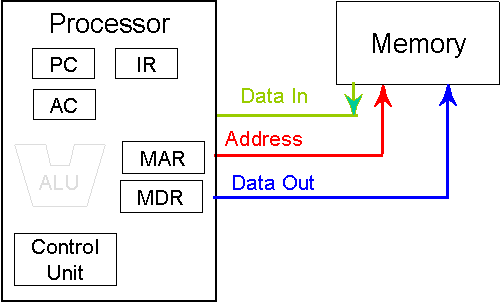PC Program counter Instruction register Accumulator Memory address register Memory data register

## Parametric Modules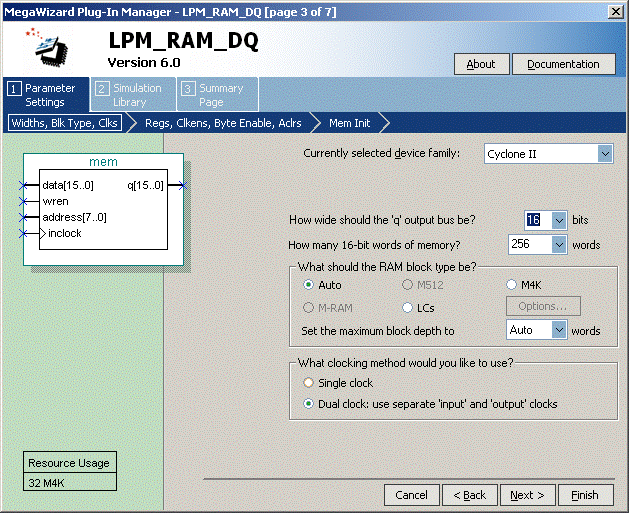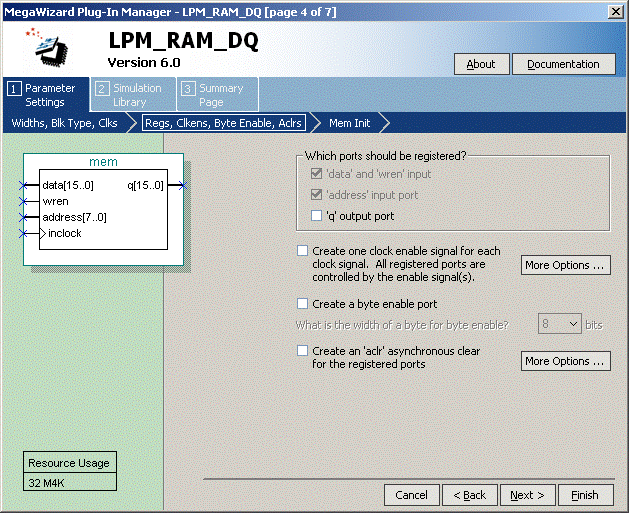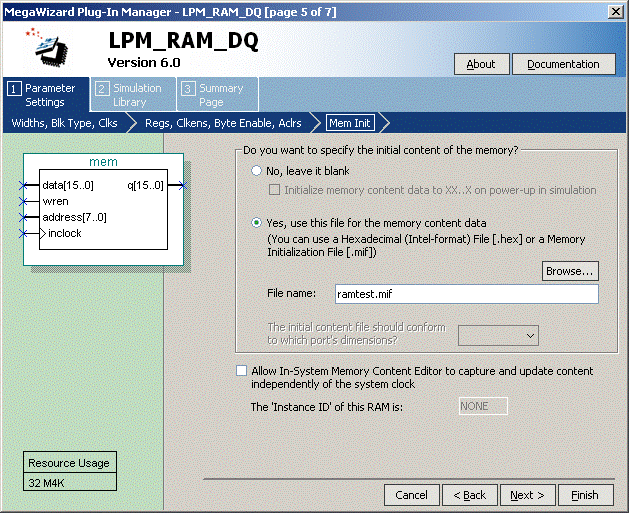## Memory Initialization File (.MIF)

```DEPTH = 256;	% Memory depth and width are required	%
WIDTH = 16;	% Enter a decimal number	%

DATA_RADIX = HEX;	% Enter BIN, DEC, HEX, or OCT; unless %
% otherwise specified, radixes = HEX	%
CONTENT
BEGIN
[00..FF]	:      0000;  % Range--00 to FF = 0000 %
00		:      0112;  % Load MEM %
01		:      0110;  % Load MEM %
02		:      0412;  % Store MEM%
03           :      0111;  % Load MEM %
04		:      0112;  % Load MEM %
05		:      0113;  % Load MEM %
10		:      0AAA;
11		:      0BBB;
12		:      0123;
13          :      0DDD;
END ;
```

## Simulation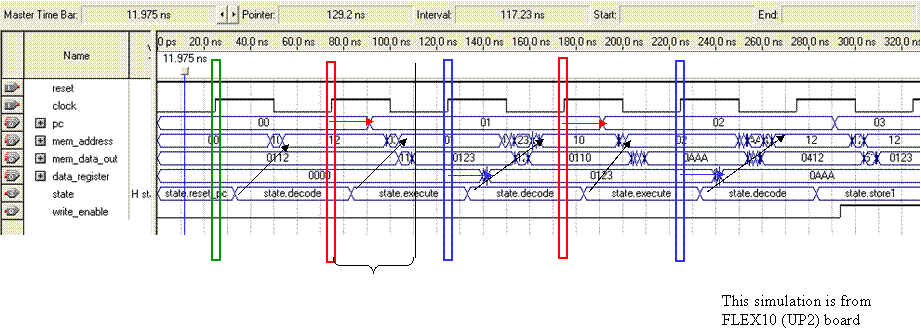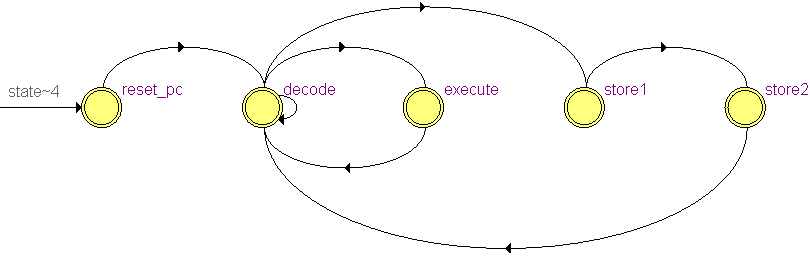```always
case (state)
endcase
```mem_address changes when state changes (combinational process)

### State Machine: reset_pc

```always @(posedge clock, negedge reset)
begin
if (~reset) state<=reset_pc;
else
case (state)
reset_pc :
begin
pc <= 8'h00;
data_register <= 16'h0000;
state <= decode;
end
```

### State Machine: decode

```// decode opcode, increment program counter
decode :
begin
pc <= pc + 8'h01;
// decode opcode
case (opcode)
state <= execute;
8'h04 : // store
state <= store1;
default:
state <= decode;
endcase
end
```

### State Machine: execute, store

```// execute instruction, save data
execute :
begin
data_register <= mem_data_out;
state <= decode;
end
store1 : state <= store2;
store2 : state <= decode;
// default state transition
default : state <= decode;
endcase
end
```

### Simulation: Store Values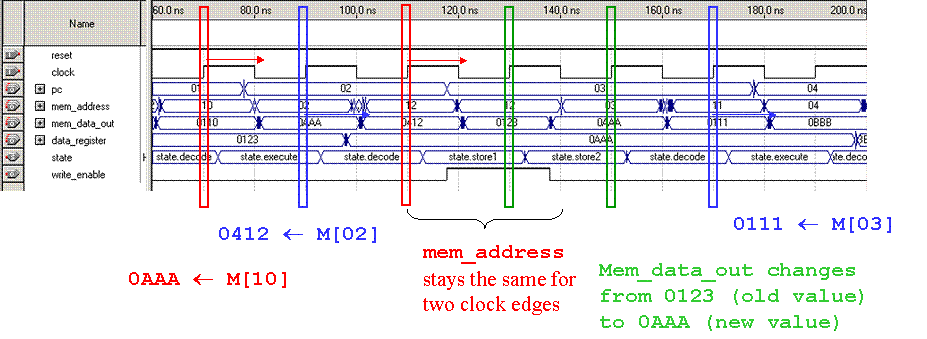assign write_enable = (state==store1);

## Verilog Code

ramtest.v

```module ramtest(clock, reset, mem_address, mem_data_out,
data_register, pc, write_enable);
input clock, reset;
output [15:0] mem_data_out;
output [15:0] data_register;
output [7:0] pc;
//output [2:0] state;
reg [2:0] state;
reg [15:0] data_register;
reg [7:0] pc;

output write_enable;

wire [7:0] opcode;
assign opcode = mem_data_out[15:8];

parameter
reset_pc = 3'd0,
decode   = 3'd1,
execute = 3'd2,
store1 = 3'd3,
store2 = 3'd4;

// instantiate memory (256 16-bit words)
mem mem_component(
.data (data_register),
.inclock (clock),
.wren (write_enable),
.q (mem_data_out)
);

//assign write_enable = (state==store1?clock:1'b0); // this fails
assign write_enable = (state==store1); // this works

always
case (state)
endcase

// finite state machine
always @(posedge clock, negedge reset)
begin
if (~reset) state<=reset_pc;
else
case (state)
reset_pc :
begin
pc <= 8'h00;
data_register <= 16'h0000;
state <= decode;
end
// decode opcode, increment program counter
decode :
begin
pc <= pc + 8'h01;
// decode opcode
case (opcode)
state <= execute;
8'h04 : // store
state <= store1;
default:
state <= decode;
endcase
end
// execute instruction, setup instruction address
execute :
begin
data_register <= mem_data_out;
state <= decode;
end
store1 : state <= store2;
store2 : state <= decode;
// default state transition
default : state <= decode;
endcase
end
endmodule```

Maintained by John Loomis, last updated 7 October 2008